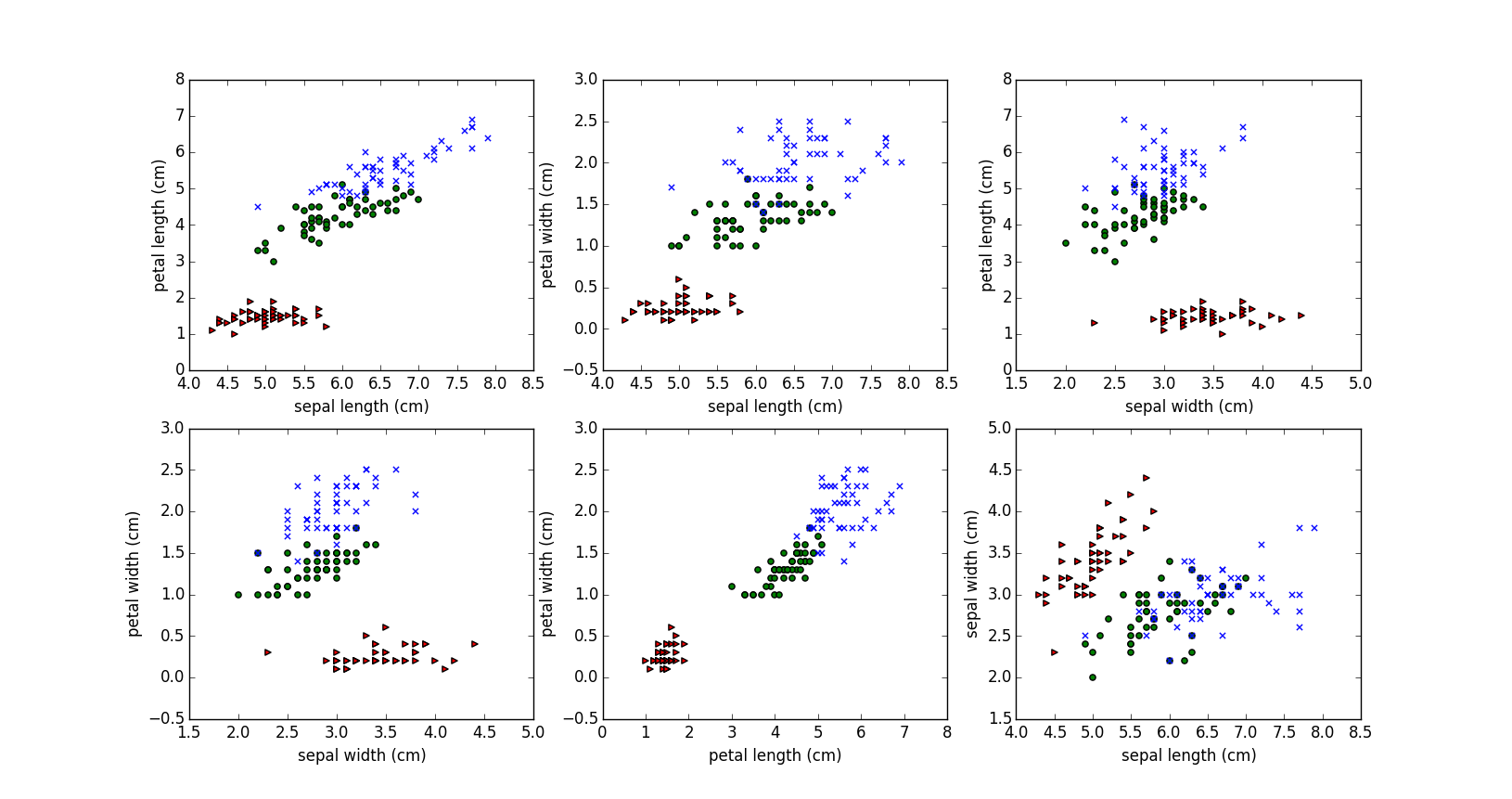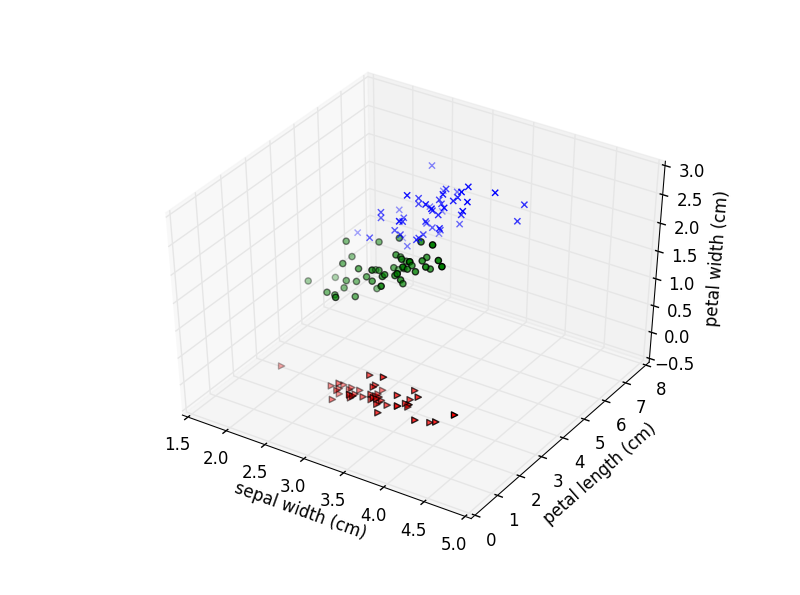## 探索sklearn | 鸢尾花数据集

1 鸢尾花数据集背景

sklearn包不仅囊括很多机器学习的算法，也自带了许多经典的数据集，鸢尾花数据集就是其中之一。

from sklearn.datasets import load_iris



找到sklearn包的路径，发现包可不少，不过现在扔在一边，以后再来探索，我现在要找到是datasets文件夹。

from .base import load_iris


2 数据的内容

150,4,setosa,versicolor,virginica
5.1,3.5,1.4,0.2,0 #花萼长度,花萼宽度,花瓣长度,花瓣宽度
4.9,3.0,1.4,0.2,0
4.7,3.2,1.3,0.2,0
4.6,3.1,1.5,0.2,0
5.0,3.6,1.4,0.2,0


第一行首先记录了样本数目150，特征数目43 数据可视化

data.keys()
>>['target_names', 'data', 'target', 'DESCR', 'feature_names']
feature = data['data'] #为numpy.ndarray类型
feature.shape #矩阵的行数和劣势
>> (150L, 4L)
target = data['target']
target.shape
>>(150L,)


四个特征是不可能同时在平面图里画出来的，只得运用我们的聪明才智，把它两两一组

def plot_iris_projection(x_index, y_index):
for t,marker,c in zip(xrange(3),'>ox', 'rgb'):
plt.scatter(data[target==t,x_index],
data[target==t,y_index],
marker=marker,c=c)
plt.xlabel(feature_names[x_index])
plt.ylabel(feature_names[y_index])pairs = [(0,1),(0,2),(0,3),(1,2),(1,3),(2,3)]
for i,(x_index,y_index) in enumerate(pairs):
plt.subplot(2,3,i)
plot_iris_projection(x_index, y_index)
plt.show()from mpl_toolkits.mplot3d import Axes3D

def plot_iris_projection3d(x_index, y_index, z_index):
fig = plt.figure()
for t,marker,c in zip(xrange(3),'>ox', 'rgb'):
ax.scatter(data[target==t,x_index],
data[target==t,y_index],
data[target==t,z_index],
marker=marker,c=c)
ax.set_xlabel(feature_names[x_index])
ax.set_ylabel(feature_names[y_index])
ax.set_zlabel(feature_names[z_index])

plot_iris_projection3d(1, 2, 3)
plt.show()posted on 2015-02-04 12:18  meelo  阅读(8462)  评论(1编辑  收藏

• 随笔 - 126
• 文章 - 0
• 评论 - 36